# Va & Weight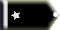n9xv Trainee

Ok, I'm a numbers guy and I came across this formula for a Piper Archer.

Manouvering speed (Va) for a given weight can be calculated as a % of the Va at max gross weight.

Their example used 2550-Lbs for max gross weight, and 1634 for the "given" weight. Va at max gross weight was 113-Kts. and their calculation came up with 92 (92.7) Kts.

So, First find the % to decrease Va by,

(1 - (given weight / max gross weight))/2

a decrease of 18-%,

113 - (.18*113) = 92.7 (92-Kts.)

My question is, does the proportional % thing apply to any aircraft (at least light single engine type anyway) as a universal formula or does it apply only to the one airplane?n9xv Trainee

For what its worth I did find out that this applies to all "conditional" speeds. The condition being weight.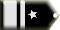Welshflyer Chief Captain

OOOHHH! That's a bit too deep for me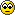Cheeks Chief Captain

lol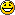VegasFlyer Chief Captain

Welshflyer wrote:

OOOHHH! That's a bit too deep for meI'm with you Welsh!Drew B (belgeode) Chief Captain

n9xv wrote:

Ok, I'm a numbers guy and I came across this formula for a Piper Archer.

Manouvering speed (Va) for a given weight can be calculated as a % of the Va at max gross weight.

Their example used 2550-Lbs for max gross weight, and 1634 for the "given" weight. Va at max gross weight was 113-Kts. and their calculation came up with 92 (92.7) Kts.

So, First find the % to decrease Va by,

(1 - (given weight / max gross weight))/2

a decrease of 18-%,

113 - (.18*113) = 92.7 (92-Kts.)

My question is, does the proportional % thing apply to any aircraft (at least light single engine type anyway) as a universal formula or does it apply only to the one airplane?

I hate math... but in answer to the original poster, it is a universal formula... only thing that changes is the numbers. Obviously different planes are going to have different thrust and weight ratios and ofcourse the maneuvering speed as a result is going to vary. You would not expect say a 737 to have the same "LISTED" maneuvering speed as your Piper Archer... cause they are different weight classes and have different power ratings for thrust.

But yeah your formula is pretty much the same for all aircraft.

Ok no more math now... my brain hurts.Welshflyer Chief Captain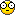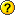WTF!All times are GMT Page 1 of 1